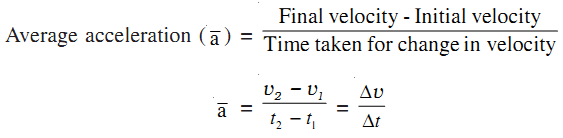The acceleration is defined as time rate of change of velocity. Acceleration is a vector quantity and its SI unit is m s–2. In one dimension, there is no need to use vector notation for acceleration.

The average acceleration of an object is given by,In one dimensional motion, when the acceleration is in the same direction as the motion or velocity (taken to be in the positive direction), the acceleration is positive. But the acceleration may be in the opposite direction of the motion also. Then the acceleration is taken as negative and is often called deceleration or retardation. An increase in the rate of change of velocity is acceleration, whereas the decrease in the rate of change of velocity is retardation.